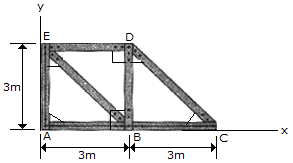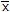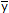# Engineering Mechanics - Center of Gravity and Centroid - Discussion

### Discussion :: Center of Gravity and Centroid - General Questions (Q.No.6)

6.The truss is made from seven members, each having a mass of 6 kg/m. Locate the position (,) of the center of mass. Neglect the mass of the gusset plates at the joints.

 [A].= 2.36 m,= 1.286 m [B].= 2.33 m,= 1.333 m [C].= 2.43 m,= 1.308 m [D].= 2.79 m,= 1.757 m

Explanation:

No answer description available for this question.

 Mathivanan said: (Oct 9, 2020) Explain the solution for this.

 Bishnu Roy said: (Jan 17, 2021) Please solve the solution.